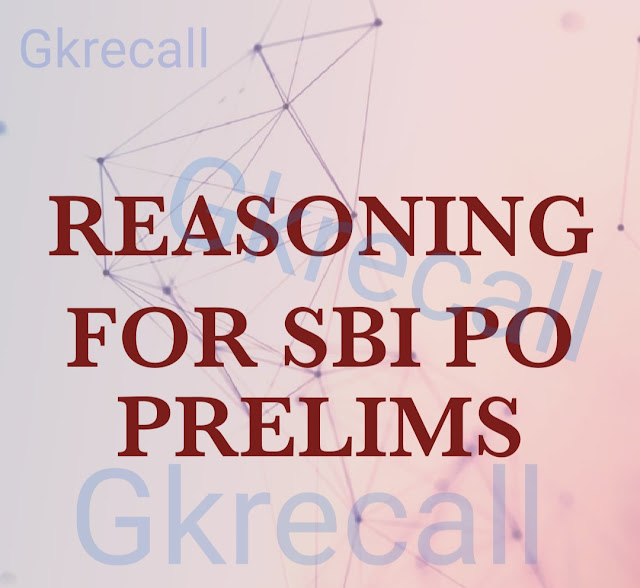# gkrecall

## BreakingWe bring you the complete and important daily Reasoning  to achieve more marks in Banking, Insurance, UPSC, SSC, CLAT, Railways and all other competitive Exams. We prepare it based on our daily current affairs.Hope you like it.

START QUIZ
Directions (1 –5): Study the following information carefully to answer the given questions
Ten boxes A, B, C, D, E, F, G, H, I and J are placed one above another but not necessarily in the same order. Three boxes are kept between Box I and box C. Box D is kept below box E. Only two boxes are kept between box C and box J. More than three boxes are kept between box J and box G, which is not kept at the top. Box G is kept above box J but not immediately above and below I. Only one box is kept between box C and G. Box I does not kept at the bottom. Box H is kept immediately above box A, which is kept above box G but not immediately above. Only three boxes are kept between box F and Box B, which is kept above box C.
1. Which of the following box is kept at the top?
A. D
B. H
C. E
D. F
E. None of these
2. How many boxes are kept between box F and box D?
A. One
B. Two
C. Three
D. None
E. None of these
3. How many boxes are kept above box I?
A. Two
B. Three
C. Four
D. None
E. None of these
4. Which of the following condition is true about box G?
A. More than four boxes are kept above box G
B. Box G is kept immediately below box E
C. Box G is kept above box F
D. More than five boxes kept below box G.
E. All are correct
5. Which of the following box is kept at the bottom?
A. C
B. D
C. F
D. E
E. None of these
Solution-
H
A
I
B
G
E
C
F
D
J
Direction: (6-10) In these questions, symbols @, #, *, \$ & © are used for different meaning as shown below:
‘A @ B’ means A is not greater than B.
‘A # B’ means A is neither greater than nor equal to B.
‘A*B’ means A is not less than B.
‘A \$ B’ means A is neither less than nor equal to B.
‘A © B’ means A is neither greater than nor less than B
6. Statement: H \$ K, K © R, R @ J, J # F
Conclusion:
I. R # H
II. F \$ R
III. H \$ J
A. Only I & II are true.
B. Only I is true.
C. Only II is true.
D. Only II & III are true.
E. All are true.
7.  Statement : L ≤ M, M * P, M # D, D \$ F
Conclusion :
I. L @ P
II. P ≤ D
III. P > D
A. Only I is true.
B. Only II is true.
C. Either I or III is true.
D. Only I & II are true.
E. None of these
8. Statement: T @ V, V # Q, Q © L, L * M
Conclusion:
I. M @ Q
II. T @ L
III. T # L
A. Only I is true.
B. Only II is true.
C. Only III is true.
D. Only I & III are true.
E. None of these
9. Statement: J * E, D @ E, E \$ K, K © T
Conclusion:
I.  J \$ D
II. J * D
III. E \$ T
A. None is true.
B. Only II & III are true.
C. Only I & III are true.
D. All are true.
E. None of these
10. Statement: H  @ I, I # L, L * A, A \$ Q.
Conclusion:
I. H # L
II. H * L
III. Q # H
A. Only I is true.
B. Only I & II are true.
C. Only III & either I or II are true.
D. Either I or III is true.
E. None of these.
Solution-
6. Option A
7. Option B
8. Option D
9. Option B
10. Option A